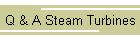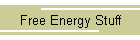Power Engineering DictionaryK

KATA THERMOMETER - Large-bulb alcohol thermometer used to measure air speed or atmospheric conditions by means of cooling effect.

KELVIN SCALE (K) - Thermometer scale on which unit of measurement equals the Celsius degree and according to which absolute zero is 0 degree, the equivalent of -273.16°C. Water freezes at 273.16 K. and boils at 373.16 K. The relationship - TK = TC + 273.16

KEROSENE - a light, hydrocarbon fuel or solvent.

KILO CALORIE - This is the amount of heat (energy) necessary to raise the temperature of 1 kg of water 1°C. (Kilo calorie = kcal)

KILO GRAIN - A unit of weight; 1000 grains.

KILOVOLT AMPERE - Product of the voltage times the current. Different from kilowatts because of inductive loads in an electrical system. Abbreviated: KVA kilo watts is equal to KVA times power factor. Kilowatt: 1000 watts. Abbreviated: kW.

KILOWATT - A measure of electrical horsepower.  A metric unit of power equal to approximately 1.34 horsepower.

KILOWATT-HOUR - A measure of electrical energy consumption. 1000 watts being consumed per hour. Abbreviated: kWh.

KINETIC ENERGY - The ability of an object to do work by virtue of its motion. (Water moving in a pipe has kinetic energy.) The energy terms that are usually used to describe the operation of a pump are "pressure" and "head". In classical mechanics, equal to one half of the body’s mass times the square of its speed.

KING VALVE - Liquid receiver (refrigeration only) service valve.

KIRCHOFF’S SECOND LAW - The law stating that, at each instant of time the increase of voltage around a close loop in a network is equal to the algebraic sum of the voltage drop.

KNOCK - In a spark ignition engine, uneven burning of the fuel/air charge that causes violent, explosive combustion and an audible metallic hammering noise. Knock results from premature ignition of the last part of the charge to burn.

KRAFT PROCESS - A wood-pulping process in which sodium sulfate is used in the caustic soda pulp-digestion liquor. Also called Kraft pulping or sulfate pulping.

kW DEMAND - The maximum rate of electric power usage required to operate a facility during a period of time, usually a month or billing period. Often called "demand".

kWh CONSUMPTION - The amount of electric energy used over a period of time; the number of kWh used per month. Often called "consumption".

KVA - Kilo Volt Amp